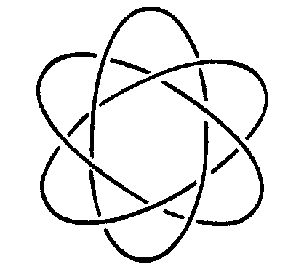# Torus knot

2010 Mathematics Subject Classification: Primary: 57K [MSN][ZBL]

of type $( p, q)$

A curve in $\mathbf R ^ {3}$ that in cylindrical coordinates $r, z, \theta$ is given by the equations

$$r = 2 + \cos t,\ \ z = \sin t,\ \ \theta = { \frac{pt }{q} } ,$$

where $t \in [ 0, 2 \pi q]$. Here $p$ and $q$ are coprime natural numbers. The torus knot lies on the surface of the unknotted torus $( r - 2) ^ {2} + z ^ {2} = 1$, intersecting the meridians of the torus at $p$ points and the parallels at $q$ points. The torus knots of types $( p, 1)$ and $( 1, q)$ are trivial. The simplest non-trivial torus knot is the trefoil (Fig. a), which is of type $( 2, 3)$. The group of the torus knot of type $( p, q)$ has a presentation $< a, b$: $a ^ {p} = b ^ {q} >$, and the Alexander polynomial is given by

$$( t ^ {pq} - 1) ( t - 1) ( t ^ {p} - 1) ^ {-} 1 ( t ^ {q} - 1) ^ {-} 1 .$$

All torus knots are Neuwirth knots (cf. Neuwirth knot). The genus of a torus knot is $( p - 1) ( q - 1)/2$.

A second construction of a torus knot uses the singularity at the origin of the algebraic hypersurface

$$V = \ \{ {( z _ {1} , z _ {2} ) \in \mathbf C ^ {2} } : { z _ {1} ^ {p} + z _ {2} ^ {q} = 0 } \} .$$

If $p$ and $q$ are coprime, then the intersection of $V$ with a sufficiently small sphere $S ^ {3} \subset \mathbf C ^ {2}$ is a knot in $S ^ {3}$ equivalent to the torus knot of type $( p, q)$. In the case when $p$ and $q$ are not coprime, this intersection also lies on an unknotted torus $T ^ {2} \subset S ^ {3}$, but consists of several components. The link so obtained is called the torus link of type $( p, q)$( cf. Fig. b, where $p = 3$, $q = 6$).Figure: t093360aFigure: t093360b